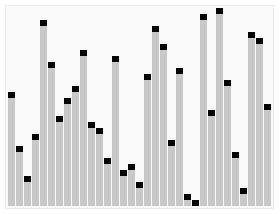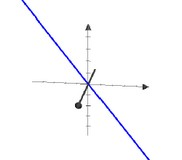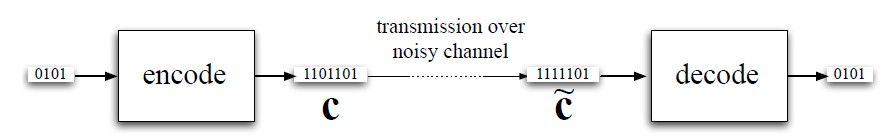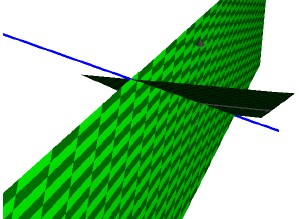# Linear Algebra - Linear Equation

A linear equation represents a linear function that forms a straight line.

A common form of a linear equation in the two variables (two dimensions) x and y is

$y= mx + b$

where:

• m is a constant named the slope or gradient of the line
• b is a constant named the intercept. It determines the point at which the line crosses the y-axis, otherwise known as the y-intercept.

In Statistics, it's the basic of a regression

## Assumptions

Terms of linear equations cannot contain:

• products of distinct or equal variables,
• nor any power (other than 1)
• or other function of a variable, equations involving terms such as xy, x2, y1/3, and sin(x)

## Linear Equation in

### Vector

In linear algebra, a linear equation can be expressed as an equation stating the value of the dot-product of:

• a coefficient vector (a vector whose entries are the coefficients)
• and a vector of unknowns variables.

Therefore a linear equation (function) is an equation of the form

$a . x = \beta$

where:

• a is a coefficient vector (for instance $1, 4, -3, \dots, 2$ ),
• x is a vector of unknowns variables (for instance $x, y, z$ or $x_1, \dots, x_n$ )
• and $\beta$ is a scalar (for instance, 3)

## Type

### Homogeneous

A linear equation a · x = 0 with zero right-hand side is a homogeneous linear equation. A system of homogeneous linear equations is called a homogeneous linear system.

## Functions

Oracle:

• Slope: REGR_SLOPE(Y,X)
• Intercept: REGR_INTERCEPT(Y,X)

## Documentation / Preference

Discover MoreAlgorithm - Linear Time - O(n)

A linear time O(n) is when the running time of an algorithm is a linear equation of its input n. ie b is not significant as it has a low level impact on the running time.ColorSpace (Model)

A color space (or model) is a coordinate space where a set of coordinate define an unique color.Geometry - Object

in Geometry An object can be: a pre-defined shape or an arbitrary one defined with the help of a path. Notions such as: The length of a vector The angle between two vectors. are introduced...Linear Algebra

“Linear” “algebra” is the branch of mathematics: concerning vector spaces, often finite or countably infinite dimensional, as well as linear mappings between such spaces. Such an investigation...Linear Algebra - (Linear system|System of Linear equations)

In mathematics, a system of linear equations (or linear system) is a collection of linear equations involving the same set of variables. Each linear system corresponds to a linear system with zero...Linear Algebra - Linear Function (Weighted sum)

f is a linear function if she is defined by where: M is an R x C matrix and A Linear function can be expressed as a matrix-vector product: If a function can be expressed as a matrix-vector product...Linear Algebra - Linear binary code

In a linear binary code, the set C of codewords is a vector space over GF(2). In such a code, there is a matrix H, called the check matrix, such that C is the null space of H. When the Receiver receives...Linear Algebra - Matrix Equation

A linear vector equation is equivalent to a matrix equation of the form : where: A is an m×n matrix, x is a column vector with n entries, and b is a column vector with m entries. A matrix...Linear Algebra - Null Space of a (Matrix|Vector Space)

Null space of a matrix A (Written Null A) is: The Null space of a matrix is a basis for the solution set of a homogeneous linear system that can then be described as a homogeneous matrix equation. A...Linear Algebra - Plane

A plane is a two dimensional vector space. A plane has a dimension of two because two coordinates are needed to specify a point on it. Two-dimensional: All points in the plane: Span {[1, 2],...